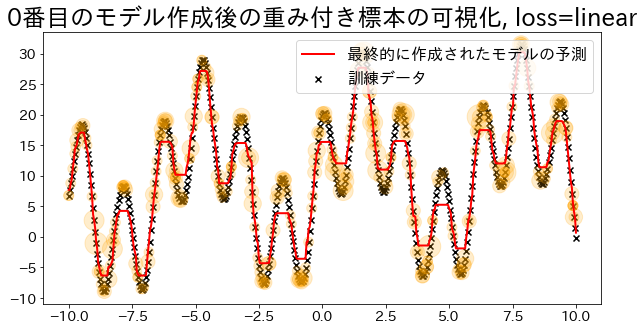import numpy as np
import matplotlib.pyplot as plt
import japanize_matplotlib
from sklearn.tree import DecisionTreeRegressor

# NOTE: This model is to check the model's sample_weight
class DummyRegressor:
def __init__(self):
self.model = DecisionTreeRegressor(max_depth=5)
self.error_vector = None
self.X_for_plot = None
self.y_for_plot = None

def fit(self, X, y):
self.model.fit(X, y)
y_pred = self.model.predict(X)

# Weights are calculated based on regression error.
# https://github.com/scikit-learn/scikit-learn/blob/main/sklearn/ensemble/_weight_boosting.py#L1130
self.error_vector = np.abs(y_pred - y)
self.X_for_plot = X.copy()
self.y_for_plot = y.copy()
return self.model

def predict(self, X, check_input=True):
return self.model.predict(X)

def get_params(self, deep=False):
return {}

def set_params(self, deep=False):
return {}


## Fit regression models to training data

# training dataset
X = np.linspace(-10, 10, 500)[:, np.newaxis]
y = (np.sin(X).ravel() + np.cos(4 * X).ravel()) * 10 + 10 + np.linspace(-2, 2, 500)

DummyRegressor(),
n_estimators=100,
random_state=100,
loss="linear",
learning_rate=0.8,
)
reg.fit(X, y)
y_pred = reg.predict(X)

# Check the fitting to the training data.
plt.figure(figsize=(10, 5))
plt.scatter(X, y, c="k", marker="x", label="訓練データ")
plt.plot(X, y_pred, c="r", label="prediction", linewidth=1)
plt.xlabel("x")
plt.ylabel("y")
plt.legend()
plt.show()## Visualize sample weights (for loss=‘linear’)

Adaboost determines the weights based on the errors of the regression. Visualize the magnitude of the weights when specified as ’linear’. Observe how data with added weights have a higher probability of being sampled during training.

loss{‘linear’, ‘square’, ‘exponential’}, default=’linear’ The loss function to use when updating the weights after each boosting iteration.

def visualize_weight(reg, X, y, y_pred):
"""Function for plotting the value (number of times sampled) corresponding to the weights of the sample

Parameters
----------
reg : sklearn.ensemble._weight_boosting
boosting model
X : numpy.ndarray
training dataset
y : numpy.ndarray
target
y_pred:
prediction
"""
assert reg.estimators_ is not None, "len(reg.estimators_) > 0"

for i, estimators_i in enumerate(reg.estimators_):
if i % 100 == 0:
# Count how many times the data appears in the data used to create the i-th model
weight_dict = {xi: 0 for xi in X.ravel()}
for xi in estimators_i.X_for_plot.ravel():
weight_dict[xi] += 1

# Plot the number of occurrences as orange circles on the graph (the more the number, the larger the circle)
weight_x_sorted = sorted(weight_dict.items(), key=lambda x: x)
weight_vec = np.array([s * 100 for xi, s in weight_x_sorted])

# plot graph
plt.figure(figsize=(10, 5))
plt.title(f"Visualization of the weighted sample after creating the {i}-th model, loss={reg.loss}")
plt.scatter(X, y, c="k", marker="x", label="training data")
plt.scatter(
estimators_i.X_for_plot,
estimators_i.y_for_plot,
marker="o",
alpha=0.2,
c="orange",
s=weight_vec,
)
plt.plot(X, y_pred, c="r", label="prediction", linewidth=2)
plt.legend(loc="upper right")
plt.show()

## Create a regression model with loss="linear
DummyRegressor(),
n_estimators=101,
random_state=100,
loss="linear",
learning_rate=1,
)
reg.fit(X, y)
y_pred = reg.predict(X)
visualize_weight(reg, X, y, y_pred)## Create regression model with loss="square
DummyRegressor(),
n_estimators=101,
random_state=100,
loss="square",
learning_rate=1,
)
reg.fit(X, y)
y_pred = reg.predict(X)
visualize_weight(reg, X, y, y_pred)